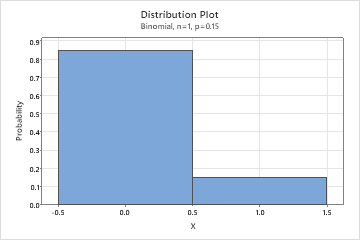# Bernoulli distribution

Use the Bernoulli distribution when a random process has exactly two outcomes—event or nonevent. For example, in the quality field, a product can be classified as good or bad.

Bernoulli variables can take two numeric values 0 or 1, where 1 corresponds to an event and 0 corresponds to a nonevent. A random variable X follows a Bernoulli distribution if, P(X = 1) = p and P(X = 0) = 1 – p, where p is the probability of an occurrence of the event.

The Bernoulli distribution is a discrete distribution that is related to many distributions, such as the binomial, geometric, and negative binomial distributions. The Bernoulli distribution represents the outcome of 1 trial. Sequences of independent Bernoulli trials generate the other distributions—the binomial distribution models the number of successes in n trials, the geometric distribution models the number of failures before the first success, and the negative binomial distribution models the number of failures before the xth success.

This plot shows a binomial distribution that has 1 trial and an event probability of 0.15. A binomial distribution with 1 trial is the same as a Bernoulli distribution.